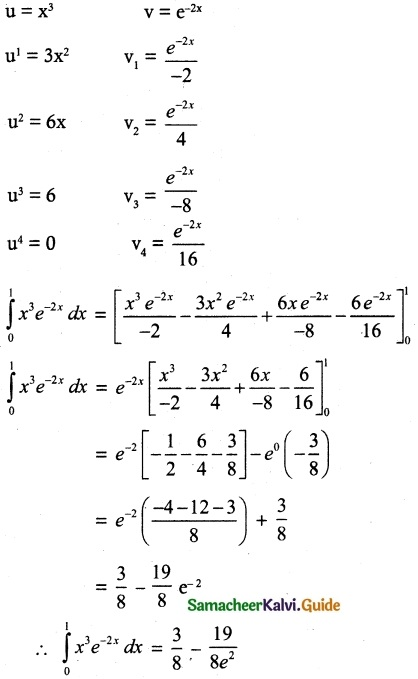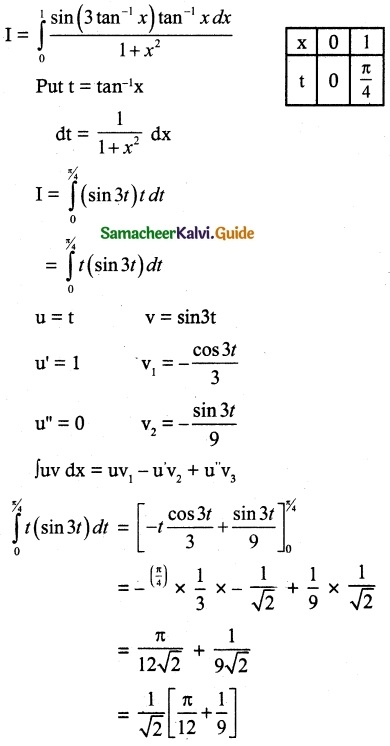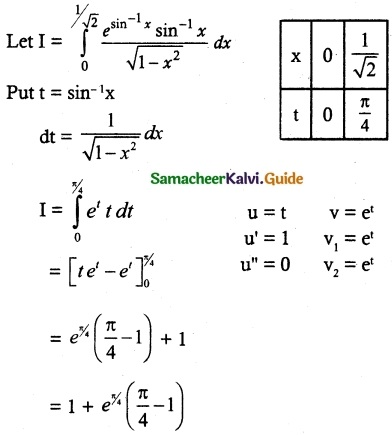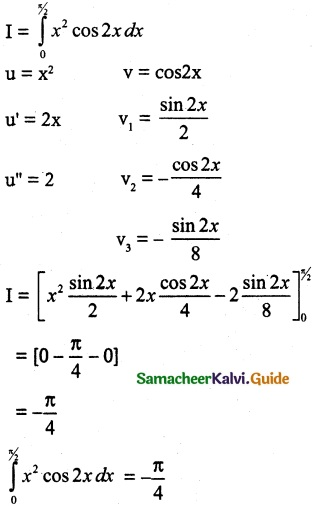Tamilnadu State Board New Syllabus Samacheer Kalvi 12th Maths Guide Pdf Chapter 9 Applications of Integration Ex 9.4 Textbook Questions and Answers, Notes.

## Tamilnadu Samacheer Kalvi 12th Maths Solutions Chapter 9 Applications of Integration Ex 9.4

Question 1.
Evaluate the following
$$\int_{0}^{1}$$ x³e-2x dx
Solution:
Bernoulli’s formula,
∫uv dx = uv1 – u1v2 + u2v3 – u3v4 + ….Question 2.
$$\int_{0}^{1}$$ $$\frac{sin(3tan^{-1}x)tan^{-1}x dx}{1+x^2}$$
Solution:Question 3.
$$\int_{0}^{1/√2}$$ $$\frac{e^{sin^{-1}x}sin^{-1}x}{\sqrt{1-x^2}}$$ dx
Solution:Question 4.
$$\int_{0}^{\pi / 2}$$ x² cos 2x dx
Solution: